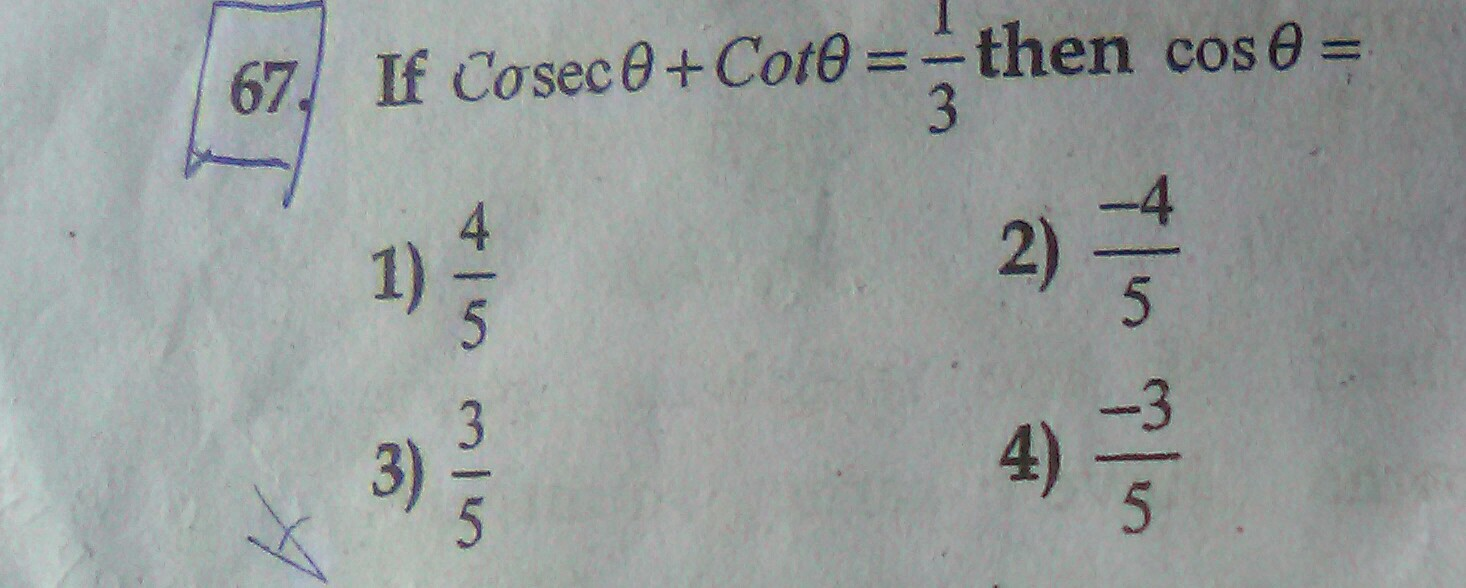# Hello please give me the answer If Cosecx+ cotx=5 then cosx=4 years ago
Dear

We start from the equation

cot A + cosec A = 3 can be written as

cos A/sin A + 1/sin A = 3, or

cos A + 1 = 3 sin A, or

cos^2 (A/2) - sin^2 (A/2) + cos^2 (A/2) + sin^2 (A/2) = 3*2 sin (A/2)cos (A/2), or

2 cos^2 (A/2) =3*2 sin (A/2)cos (A/2), or

cos (A/2) = 3sin (A/2), or

tan (A/2) = 1/3

tan A = 2tan (A/2)/[1 - tan^2 A]

= 2*(1/3)/(1 - 1/9)

=(2/3)/(8/9), or

=2*9/3*8

= 3/4

So Pythagoras comes in here, height of right-angled triangle is 3, its base is 4, hence the hypotenuse is 5, therefore

cos A = 4/5 and sin A = 3/5